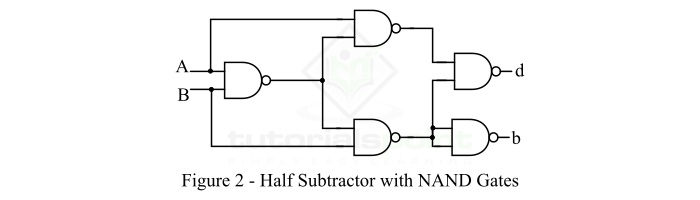# Half Subtractor Using NAND Gates

In digital electronics, a subtractor is a combinational logic circuit that performs the subtraction of two binary numbers. However, the subtraction of binary number can be performed using adder circuits by taking 1’s or 2’s compliments. But, we may also realize a dedicate circuit to perform the subtraction of two binary numbers.

In the subtraction of two binary numbers, each subtrahend bit of the number is subtracted from its corresponding significant minuend bit to form a difference bit. During the subtraction, if the minuend bit is smaller than the subtrahend bit, then a 1 is borrowed from the next position. Depending upon the number of bits taken as input, there are two types of subtractors namely, Half Subtractor and Full Subtractor.

A half subtractor is one which takes two binary digits as input and gives a difference bit and a borrow bit (if any) as output.

On the other hand, a full subtractor is one that takes three bits as input, i.e. two are the input bits and one is the input borrow bit from the previous stage, and gives a difference bit and a output borrow bit as the output.

Since a subtractor is a combinational logic circuit, i.e. it is made of logic gates. We can realize a full adder circuit using different types of logic gates like AND, OR, NOT, NAND, NOR, etc.

In this article, we will discuss the implementation of a half subtractor using NAND gates. But before that let’s have a look into the basics of the half subtractor.

## What is Half Subtractor?

A half-subtractor is a combinational circuit which has two inputs and two outputs where one output is difference and another is borrow bit. The half subtractor produces the difference between the two binary bits at the input and also produces a borrow output (if any). In the subtraction (A-B), A is called as Minuend bit and B is called as Subtrahend bit. The block diagram of the half subtractor is shown in Figure-1.Here, A and B are the input variables (binary digits) and d is the output difference bit and b is the borrow bit. We can understand the operation of a half subtractor with the help of its truth table.

## Truth Table of Half Subtractor

The following is the truth table the half subtractor −

Inputs Outputs
A B d (Difference) b (Borrow)
0 0 0 0
0 1 1 1
1 0 1 0
1 1 0 0

Using this truth table, we can determine the output equation of the half subtractor. The following are the equations of difference bit (d) and borrow bit (b) −

Different Bit (d) −

$$\mathrm{Difference,\, d=A'B+AB'=A\oplus B}$$

Borrow Bit (b)

$$\mathrm{Borrow,\, b=A'B}$$

Now, let us discuss the realization of half subtractor using NAND gates.

## Half Subtractor Using NAND Gates

We may implement the logic circuit of half subtractor using NAND gates only as shown in figure-2.From this logic circuit diagram, we can see that 9 NAND gates are required for realization of the half subtractor.

The output equations of the half subtractor in NAND logic are as follows −

Difference Bit (d)

$$\mathrm{Difference,\, d=\overline{\overline{A\cdot \overline{AB}}\cdot \overline{B\cdot \overline{AB}}}=A\oplus B}$$

Borrow Bit (b)

$$\mathrm{Borrow,\, b=\overline{\overline{B\cdot \overline{AB}}}=\overline{A}B}$$

In this way, we can realize the half subtractor using the NAND gates only.

Updated on: 26-Dec-2022

4K+ Views